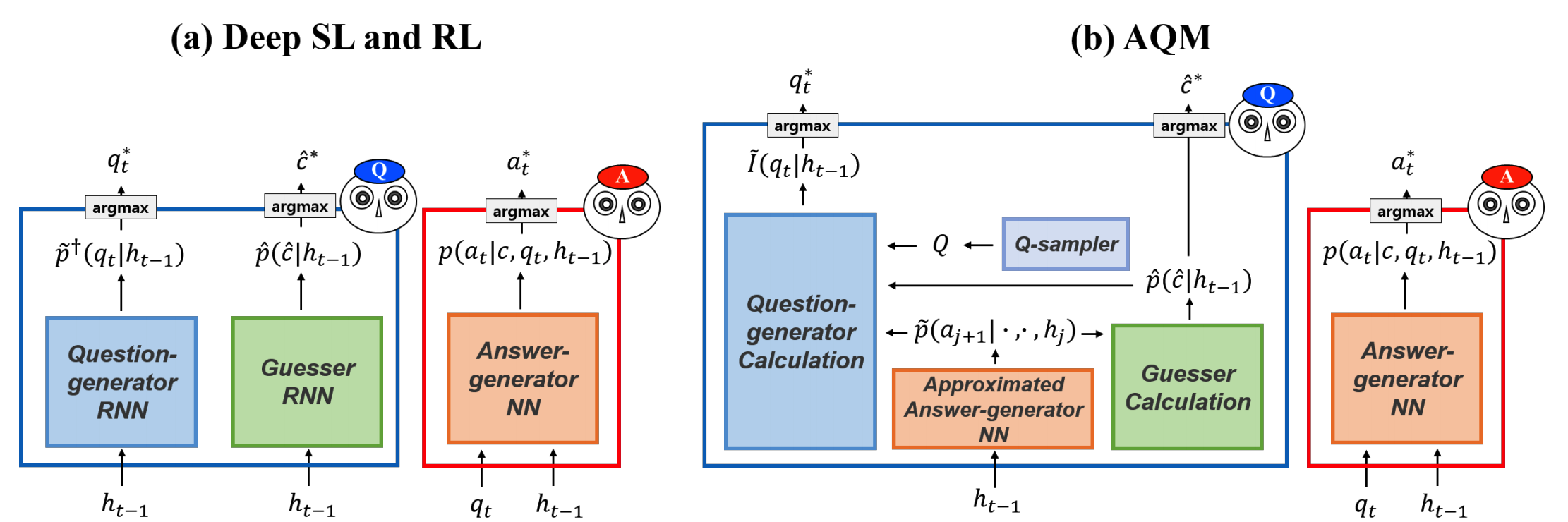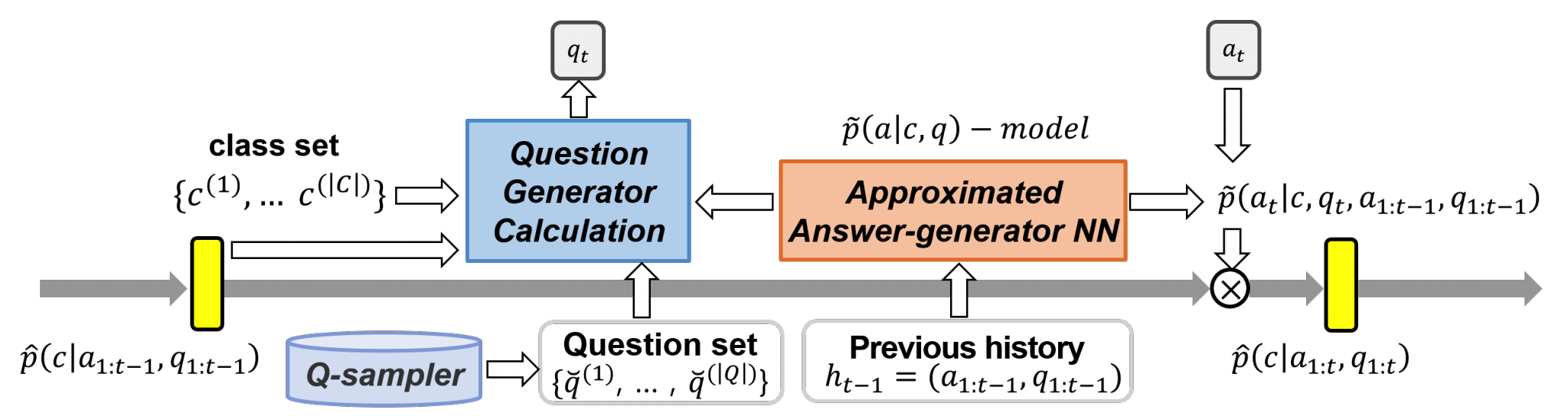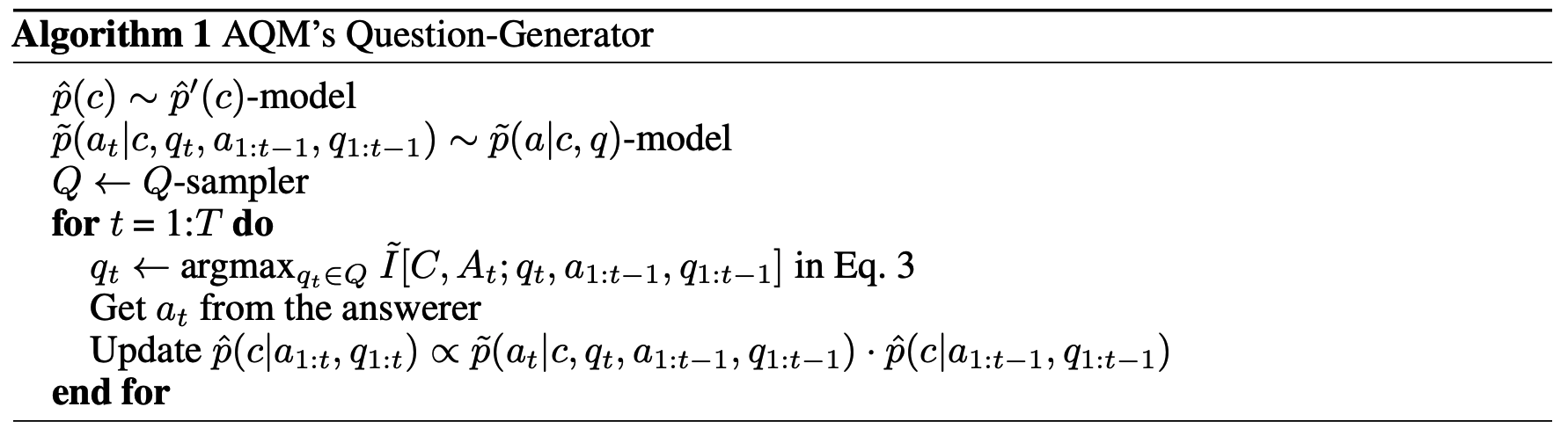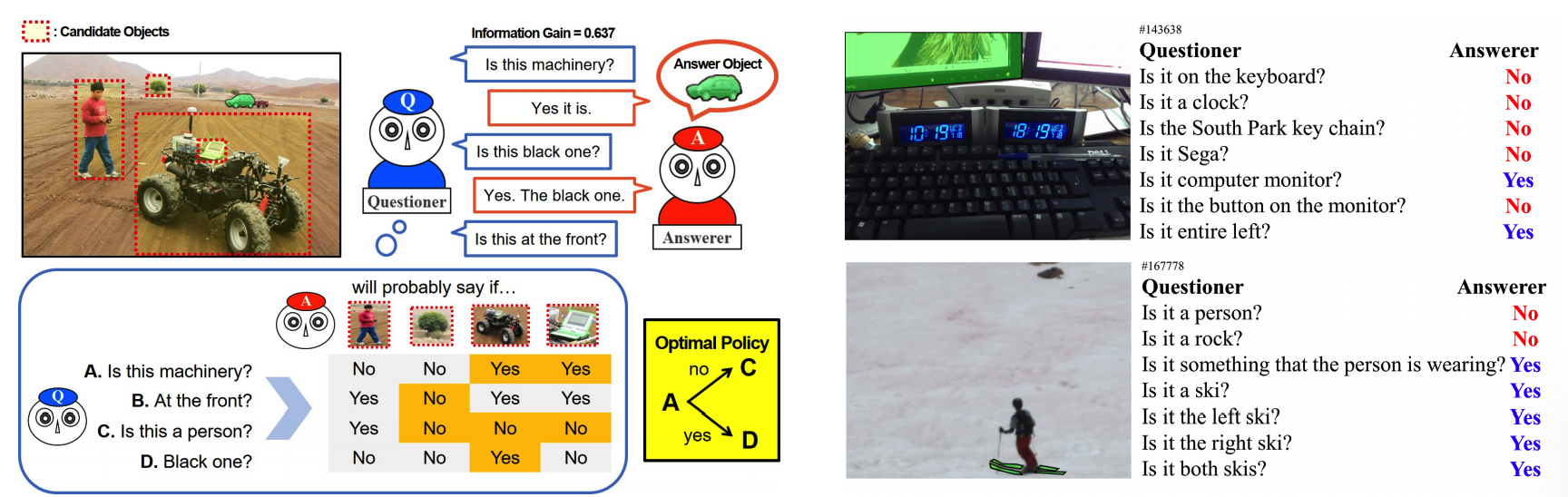# Answerer in Questioner's Mind: Information Theoretic Approach to Goal-Oriented Visual Dialog

## WHY?

Goal-oriented dialogue tasks require two agents(a questioner and an answerer) to communicate to solve the task. Previous supervised learning or reinforcement learning approaches struggled to make appropriate question due to the complexity of forming a sentence. This paper suggests information theoretic approach to solve this task.

## WHAT?The answerer model for VQA is a simple neural network model which is the same as previous methods. In former SL and RL methods, questioner had two RNN-based models, one for generating a question and one for guessing the answer image. On the other hand, the questioner of AQM uses mathmatical calculation instead of RNN models.The questioner of AQM first generates question candidates to choose from (Q-sampler). Next, the questioner calculate the information gain for each of question candidate and choose the question with the greatest information gain.

I[C, A_t; q_t, a_{1:t-1}, q_{1:t-1}]\\
= H[C; a_{1:t-1}, q_{1:t-1}] - H[C|A_t; q_t, a_{1:t-1}, q_{1:t-1}]\\
= \sum_{a_t}\sum_c p(c|a_{1:t-1}, q_{1:t-1})p(a_t|c, q_t, a_{1:t-1}, q_{1:t-1}) \ln \frac{p(a_t|c, q_t, a_{1:t-1}, q_{1:t-1})}{p(a_t|q_t, a_{1:t-1}, q_{1:t-1})}\\
p(c|a_{1:t}, q_{1:t}) \propto p(c)\prod_{j=1}^t p(a_j|c, q_j, a_{1:j-1}, q_{1:j-1})

\hat{p}(a_t|c, q_t, a_{1:t-1}, q_{1:t-1}) \propto \tilde{p}'(c)\prod_{j=1}^t \tilde{p}(a_j|c, q_j, a_{1:j-1}, q_{1:j-1})

The questioner selects the question that maximize the information gain based on this approximate answer distribution posterior.

q_t^* = argmax_{q_t \in Q} \tilde{I}[C, A_t; q_t, a_{1:t-1}, q_{1:t-1}]\\
= argmax_{q_t \in Q} \sum_{a_t}\sum_c \hat{p}(c|a_{1:t-1}, q_{1:t-1})\tilde{p}(a_t|c, q_t, a_{1:t-1}, q_{1:t-1}) \ln \frac{\tilde{p}(a_t|c, q_t, a_{1:t-1}, q_{1:t-1})}{\tilde{p}'(a_t|q_t, a_{1:t-1}, q_{1:t-1})}\\
\tilde{p}'(a_t|q_t, a_{1:t-1}, q_{1:t-1}) = \sum_c\hat{p}(c|a_{1:t-1}, q_{1:t-1})\cdot\tilde{p}(c, q_t, a_{1:t-1}, q_{1:t-1})

The algorithm for AQM’s questioner is as follows.In practice, there are some options for implementation. First, Q-sampler that samples question candidates can be randQ that sample questions at random or countQ that sample questions with the least correlation. Second, YOLO9000 is used to pick the list of candidate objects and the prior for these candidate is set to 1/N. Third, the answerer model picks the answer independent of the history. On the other hand, the approximate for the answerer model can be varied. The approximate for the answerer model can be trained independently from answerer model(indA) or trained from the answer from answerer model(depA). While these two share the same training datatset, indAhalf and depAhalf do not share the dataet.

## So?AQM outperformed previous methods in goal oriented dialogue tasks such as MNIST Counting Dialog and GuessWhat?!. GuessWhat?! is one of the goal-oriented dialogue tasks that the questioner needs to guess the object in an image by asking questions to the answerer while only the answerer knows the answer.

Powered by aiden